Some details for Foundations of cosmology

ANIL MITRA, COPYRIGHT © FEBRUARY 2016 – June 2017

Home

Contents

General relativity: field equations

Quantum theory: some equations

Schrödinger:

Klein-Gordon

Dirac: Lorentz Invariant form

Pauli

Quantum electrodynamics

Quantum chromodynamics

Some details for roundations of cosmology

# General relativity: field equations

With thanks to Wikipedia.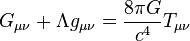Gμν is symmetric:where gμν is the metric tensor.

The curvature scalar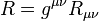The Ricci tensor, Rμν is related to the more general Riemann curvatureTμν is the energy momentum tensor.

# Quantum theory: some equations

With thanks to Wikipedia.

## Schrödinger:## Klein-Gordon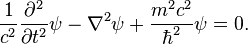## Dirac: Lorentz Invariant form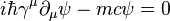## Pauli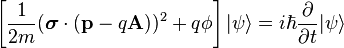## Quantum electrodynamics

The Lagrangian for a spin-1/2 field interacting with the electromagnetic field is given by the real part of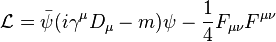whereare Dirac matrices;a bispinor field of spin-1/2 particles (e.g. electron–positron field);, called "psi-bar", is sometimes referred to as the Dirac adjoint;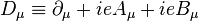is the gauge covariant derivative;

e is the coupling constant, equal to the electric charge of the bispinor field;

m is the mass of the electron or positron;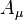is the covariant four-potential of the electromagnetic field generated by the electron itself;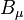is the external field imposed by external source;is the electromagnetic field tensor.

## Quantum chromodynamics

The Lagrangian density is: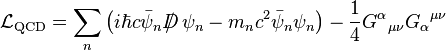D is the QCD gauge invariant derivative, n = 1 … 6, counts the quark types and Gαμν is the gluon field strength tensor.